## Wednesday, November 13, 2013

### ggplot2: Cheatsheet for Scatterplots

Quick Intro to ggplot2

The graphics package ggplot2 is powerful, aesthetically pleasing, and (after a short learning curve to understand the syntax) easy to use. I have made some pretty cool plots with it, but on the whole I find myself making a lot of the same ones, since doing something over and over again is generally how research goes. Since I constantly forget the options that I need to customize my plots, this next series of posts will serve as cheatsheets for scatterplots, barplots, and density plots. We start with scatterplots.

### Quick Intro to ggplot2

The way ggplot2 works is by layering components of your plot on top of each other. You start with the basic of the data you want your plot to include (x and y variables), and then layer on top the kind of plotting colors/symbols you want, the look of the x- and y-axes, the background color, etc. You can also easily add regression lines and summary statistics.

For great reference guides, use the ggplot2 documentation or the R Graphs Cookbook.

In this post, we focus only on scatterplots with a continuous x and continuous y. We are going to use the mtcars data that is available through R.

``````library(ggplot2)
library(gridExtra)
mtc <- mtcars
``````

Here's the basic syntax of a scatterplot. We give it a dataframe, mtc, and then in the aes() statement, we give it an x-variable and a y-variable to plot. I save it as a ggplot object called p1, because we are going to use this as the base and then layer everything else on top:

``````# Basic scatterplot
p1 <- ggplot(mtc, aes(x = hp, y = mpg))
``````

Now for the plot to print, we need to specify the next layer, which is how the symbols should look - do we want points or lines, what color, how big. Let's start with points:

``````# Print plot with default points
p1 + geom_point()
``````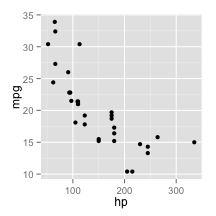That's the bare bones of it. Now we have fun with adding layers. For each of the examples, I'm going to use the grid.arrange() function in the gridExtra package to create multiple graphs in one panel to save space.

### >> Change color of points

We start with options for colors just by adding how we want to color our points in the geom_point() layer:

``````p2 <- p1 + geom_point(color="red")            #set one color for all points
p3 <- p1 + geom_point(aes(color = wt))        #set color scale by a continuous variable
p4 <- p1 + geom_point(aes(color=factor(am)))  #set color scale by a factor variable

grid.arrange(p2, p3, p4, nrow=1)
``````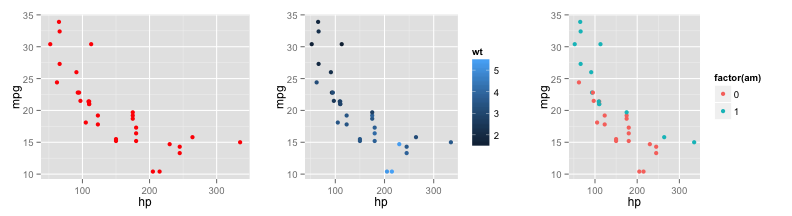We can also change the default colors that are given by ggplot2 like this:

``````#Change default colors in color scale
p1 + geom_point(aes(color=factor(am))) + scale_color_manual(values = c("orange", "purple"))
``````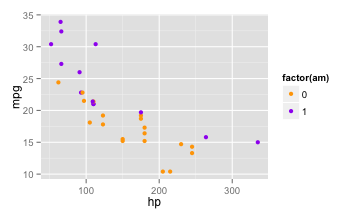### >> Change shape or size of points

We're sticking with the basic p1 plot, but now changing the shape and size of the points:

``````p2 <- p1 + geom_point(size = 5)                   #increase all points to size 5
p3 <- p1 + geom_point(aes(size = wt))             #set point size by continuous variable
p4 <- p1 + geom_point(aes(shape = factor(am)))    #set point shape by factor variable

grid.arrange(p2, p3, p4, nrow=1)
``````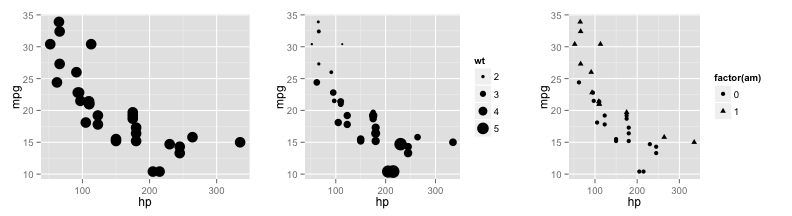Again, if we want to change the default shapes we can:

``````p1 + geom_point(aes(shape = factor(am))) + scale_shape_manual(values=c(0,2))
``````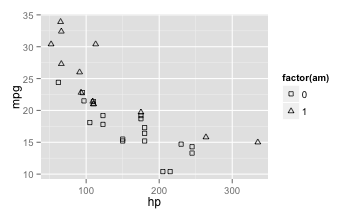### >> Add lines to scatterplot

``````p2 <- p1 + geom_point(color="blue") + geom_line()                           #connect points with line
p3 <- p1 + geom_point(color="red") + geom_smooth(method = "lm", se = TRUE)  #add regression line
p4 <- p1 + geom_point() + geom_vline(xintercept = 100, color="red")         #add vertical line

grid.arrange(p2, p3, p4, nrow=1)
``````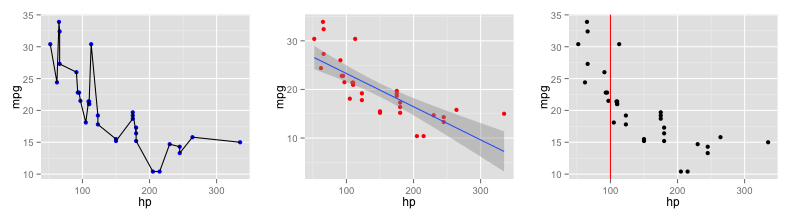You can also take out the points, and just create a line plot, and change size and color as before:

``````ggplot(mtc, aes(x = wt, y = qsec)) + geom_line(size=2, aes(color=factor(vs)))
``````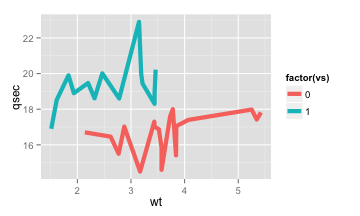### >> Change axis labels

There are a few ways to do this. If you only want to quickly add labels you can use the labs() layer. If you want to change the font size and style of the label, then you need to use the theme() layer. More on this at the end of this post. If you want to change around the limits of the axis, and exactly where the breaks are, you use the scale_x_continuous (and scale_y_continuous for the y-axis).

``````p2 <- ggplot(mtc, aes(x = hp, y = mpg)) + geom_point()

p3 <- p2 + labs(x="Horsepower",
y = "Miles per Gallon")                                  #label all axes at once

p4 <- p2 + theme(axis.title.x = element_text(face="bold", size=20)) +
labs(x="Horsepower")                                          #label and change font size

p5 <- p2 + scale_x_continuous("Horsepower",
limits=c(0,400),
breaks=seq(0, 400, 50))                    #adjust axis limits and breaks

grid.arrange(p3, p4, p5, nrow=1)
``````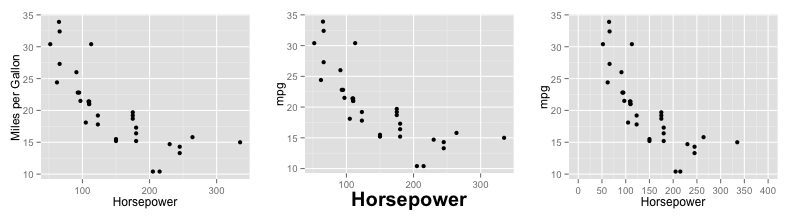### >> Change legend options

We start off by creating a new ggplot base object, g1, which colors the points by a factor variable. Then we show three basic options to modify the legend.

``````g1<-ggplot(mtc, aes(x = hp, y = mpg)) + geom_point(aes(color=factor(vs)))

g2 <- g1 + theme(legend.position=c(1,1),legend.justification=c(1,1))        #move legend inside
g3 <- g1 + theme(legend.position = "bottom")                                #move legend bottom
g4 <- g1 + scale_color_discrete(name ="Engine",
labels=c("V-engine", "Straight engine"))    #change labels

grid.arrange(g2, g3, g4, nrow=1)
``````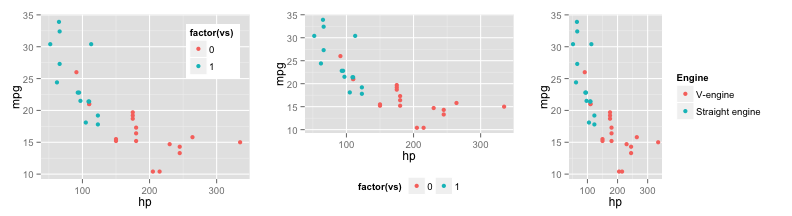If we had changed the shape of the points, we would use scale_shape_discrete() with the same options. We can also remove the entire legend altogether by using theme(legend.position=“none”)

Next we customize a legend when the scale is continuous:

``````g5<-ggplot(mtc, aes(x = hp, y = mpg)) + geom_point(size=2, aes(color = wt))
g5 + scale_color_continuous(name="Weight",                                     #name of legend
breaks = with(mtc, c(min(wt), mean(wt), max(wt))), #choose breaks of variable
labels = c("Light", "Medium", "Heavy"),            #label
low = "pink",                                      #color of lowest value
high = "red")                                      #color of highest value
``````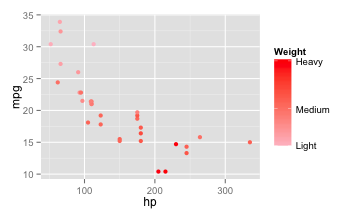### >> Change background color and style

The look of the plot in terms of the background colors and style is the theme(). I personally don't like the look of the default gray so here are some quick ways to change it. I often the theme_bw() layer, which gets rid of the gray.

``````g2<- ggplot(mtc, aes(x = hp, y = mpg)) + geom_point()

#Completely clear all lines except axis lines and make background white
t1<-theme(
plot.background = element_blank(),
panel.grid.major = element_blank(),
panel.grid.minor = element_blank(),
panel.border = element_blank(),
panel.background = element_blank(),
axis.line = element_line(size=.4)
)

#Use theme to change axis label style
t2<-theme(
axis.title.x = element_text(face="bold", color="black", size=10),
axis.title.y = element_text(face="bold", color="black", size=10),
plot.title = element_text(face="bold", color = "black", size=12)
)

g3 <- g2 + t1
g4 <- g2 + theme_bw()
g5 <- g2 + theme_bw() + t2 + labs(x="Horsepower", y = "Miles per Gallon", title= "MPG vs Horsepower")

grid.arrange(g2, g3, g4, g5, nrow=1)
``````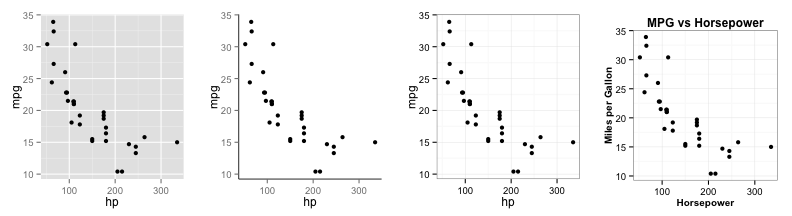Finally, here's a nice graph using a combination of options:

``````g2<- ggplot(mtc, aes(x = hp, y = mpg)) +
geom_point(size=2, aes(color=factor(vs), shape=factor(vs))) +
geom_smooth(aes(color=factor(vs)),method = "lm", se = TRUE) +
scale_color_manual(name ="Engine",
labels=c("V-engine", "Straight engine"),
values=c("red","blue")) +
scale_shape_manual(name ="Engine",
labels=c("V-engine", "Straight engine"),
values=c(0,2)) +
theme_bw() +
theme(
axis.title.x = element_text(face="bold", color="black", size=12),
axis.title.y = element_text(face="bold", color="black", size=12),
plot.title = element_text(face="bold", color = "black", size=12),
legend.position=c(1,1),
legend.justification=c(1,1)) +
labs(x="Horsepower",
y = "Miles per Gallon",
title= "Linear Regression (95% CI) of MPG vs Horsepower by Engine type")

g2
``````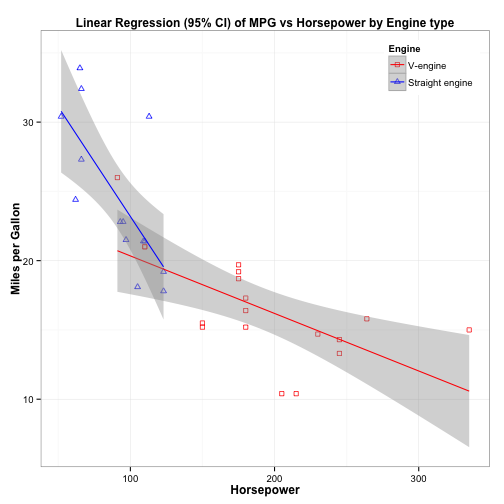### >> Reader request: Display Regression Line Equation on Scatterplot

I received a request asking how to overlay the regression equation itself on a plot, so I've decided to update this post with that information.

There are two ways to put text on a ggplot: annotate or geom_text(). I was finding that the geom_text() layer did not look very nice on my screen so I checked up on it and it seems others have this issue as well. I'll show you how the two behave, at least in my version of everything I use on my mac.

We'll go back to the example where I add a regression line to the plot using geom_smooth(). To add text, you need to run the regression outside of ggplot, extract the coefficients, and then paste them together into some text that you can layer onto the plot.

We're plotting MPG against horsepower so we create an object m that stores the linear model, and then extract the coefficients using the coef() function. We envelope the coef() function with signif() in order to round the coefficients to two significant digits. I then paste the regression equation text together, using sep=“” in order to eliminate spaces.

``````m <- lm(mtc\$mpg ~ mtc\$hp)
a <- signif(coef(m), digits = 2)
b <- signif(coef(m), digits = 2)
textlab <- paste("y = ",b,"x + ",a, sep="")
print(textlab)
``````
``````##  "y = -0.068x + 30"
``````

Next, I take the original p1 ggplot object, add points and a linear model to it, and then add a layer of text. I will show the two ways here, first using geom_smooth and then using annotate.

With both methods, you must specify the x- and y-coordinates for where the text should be centered. In the geom_text code, notice that that label=textlab is included in the aes statement, while this is not the case for annotate. If there were mathematical or formatting symbols in the text, I would indicate parse=TRUE instead of FALSE, as we will see in the next example.

``````##basic ggplot with points and linear model
p3 <- p1 + geom_point(color="red") + geom_smooth(method = "lm", se = TRUE)

r1 <- p3 + geom_text(aes(x = 245, y = 30, label = textlab), color="black", size=5, parse = FALSE)

r2 <- p3 + annotate("text", x = 245, y = 30, label = textlab, color="black", size = 5, parse=FALSE)

grid.arrange(r1, r2, nrow=1)
``````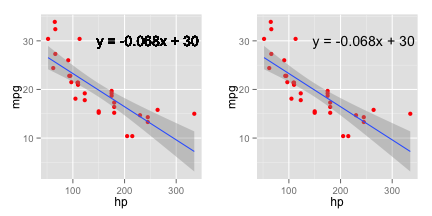In a fancier way that I got from this StackOverflow page, you can use a function to piece together your text (which would be useful if you were doing this a lot). It also shows you how you can put in mathematical symbols and formattting changes, like making your variables italic by using substitute(), and adding in a dot for the multiplication symbol.

The function lm_eqn() takes the arguments x, y, and a dataframe and evaluates the same linear model as before. Then it uses the substitute() function to piece together the regression equation using an expression, which is an R object of class “call”.

Finally, the function returns the expression, and is used exactly the same way in the two ggplot statements, EXCEPT that since we now have these formatting changes, we must use parse=TRUE in order to properly display the expressions.

``````##function to create equation expression
lm_eqn = function(x, y, df){
m <- lm(y ~ x, df);
eq <- substitute(italic(y) == b %.% italic(x) + a,
list(a = format(coef(m), digits = 2),
b = format(coef(m), digits = 2)))
as.character(as.expression(eq));
}

r3 <- p3 + geom_text(aes(x = 245, y = 30, label = lm_eqn(mtc\$hp, mtc\$mpg, mtc)), color="black", size=5, parse = TRUE)

r4 <- p3 + annotate("text", x = 245, y = 30, label = lm_eqn(mtc\$hp, mtc\$mpg, mtc), color="black", size = 5, parse=TRUE)

grid.arrange(r3, r4, nrow=1)
``````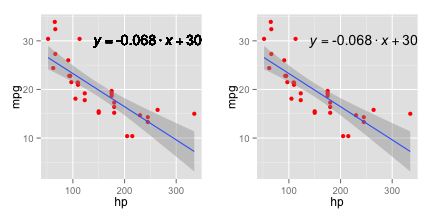Of course, you can change the font and do more formatting stuff on the text itself - find that information here.

Lastly, I will go over functions in a post that I plan on doing very soon so be on the lookout for that if the function used here is confusing or you'd like to know more.

1.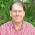2.Indeed, very clear and helpful. One question: in your last example, you change both colour and shape to vary with vs. Having colour represent vs, and shape, say, am, is not a problem; but how does one construct a suitable legend?

1.Thanks! You would change scale_shape_manual and scale_color_manual accordingly. I took out the regression lines because it would be confusing but here is the plot with color by vs and shape by am with the legend:

g2<- ggplot(mtc, aes(x = hp, y = mpg)) +
geom_point(size=3, aes(color=factor(vs), shape=factor(am))) +
scale_color_manual(name ="Engine",
labels=c("V-engine", "Straight"),
values=c("red","blue")) +
scale_shape_manual(name ="Transmission",
labels=c("Automatic", "Manual"),
values=c(0,2)) +
theme_bw() +
theme(
axis.title.x = element_text(face="bold", color="black", size=12),
axis.title.y = element_text(face="bold", color="black", size=12),
plot.title = element_text(face="bold", color = "black", size=12),
legend.position=c(1,1),
legend.justification=c(1,1)) +
labs(x="Horsepower", y = "Miles per Gallon", title= "MPG vs Horsepower by Engine and Transmission")

2.Works like a charm. Thanks!

3.Some of the plots are not loading (e.g. 4, 6, 8, 10, ...)

4.Hmm, they look fine to me. Which one specifically doesn't load? Or can you send me a screenshot?
srokicki@fas.harvard.edu

3.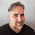4.5.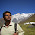Hi Rokicki.. I'm also Public Health researcher and admire R very much. Its amazing to learn more of R from your blog. I liked this particular ggplot series on Scatterplot.. I would like to know how we can put the regression equation onto the plot, for example in your plot
p3 <- p1 + geom_point(color="red") + geom_smooth(method = "lm", se = TRUE) #add regression line

Thank you.

1.Hi Manoj, Great question! I have updated the Scatterplot blog post to answer it. Check out the last section now and I hope it helps! Thanks for reading.

6.Thanks for sharing, that what useful. However, annotate() is a better way than geom_text(), as you can see from the poor, jagged annotations it produces, caused by printing over and over. See http://stackoverflow.com/questions/11618392/ggplot-text-printed-by-geom-text-is-not-clear

7.Thank you very much for taking the initiative to organize this very useful information in a clear and concise way.

I recently finished MITx's excellent 15.071x MOOC in data analytics, and this post plus your

http://www.r-bloggers.com/ggplot2-cheatsheet-for-visualizing-distributions/

complement the visualization unit of that course very well.

1.Thanks Nick! I'm really glad it's helpful. That class sounds really interesting. I'll check it out.

8.I love this!